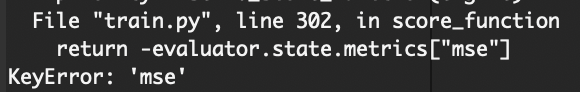# Nested event handlers

What if I want to run my evaluation engine (which itself has event handlers) with my evaluation dataloader every 20 epochs of the training engine? Right now this isn’t possible, so I made a wrapper function that allows for this below. However, I was wondering if there was an ignite way of doing this? I couldn’t find any.

``````def _lf_two(
log_fn: Callable[[Engine, List[str]], None],
inner_engine: Engine,
**kwargs
) -> Callable[[Engine], Any]:
"""Returns a lambda calling custom log function with two engines (e.g. the training loop and validation loop)"""
return lambda outer_engine: log_fn(
)

def run_engine_and_log_output(
) -> None:

###Here i grab things from engine.state.output and do what i want###

Events.EPOCH_COMPLETED(every=20),
_lf_two(
run_engine_and_log_output,
evaluator,
``````

Also, I was wondering if I then wanted to run the evaluation outside of the training loop, and this time with different event handlers, would best practice be to create two different evaluation engines? One to pass into the training loop event handler into (__lf_two()), and one to run after my train.run ?

Thanks!

Maybe I do not correctly understand the issue, but if you would like to run model evaluation every 20-th epochs and in the end of the training it is simply done like :

``````
trainer = ...
evaluator = ...

@trainer.on(Events.EPOCH_COMPLETED(every=20) | Events.COMPLETED)
def validate_model():
print(trainer.state.epoch, state.metrics)

``````

Well sort of - I want to do :

handler = ModelCheckpoint(
“models/”,
“checkpoint”,
score_function=score_function,
n_saved=None
)

except I want it to be the EPOCH_COMPLETED(every=50) of the training epoch, not the evaluator epoch…

except I want it to be the EPOCH_COMPLETED(every=50) of the training epoch, not the evaluator epoch…

It means that you would like to save a model (not the best one) every 50-th epoch completed. This is easy following the doc of ModelCheckpoint

``````handler = ModelCheckpoint(“models/”, “checkpoint”, n_saved=None)

``````

so, here is a complete working example

``````!rm -rf /tmp/models

import os
from ignite.engine import Engine, Events
from ignite.handlers import ModelCheckpoint
from torch import nn
trainer = Engine(lambda engine, batch: None)
handler = ModelCheckpoint('/tmp/models', 'myprefix', n_saved=None, create_dir=True)
model = nn.Linear(3, 3)
trainer.run(, max_epochs=200)

!ls /tmp/models
``````

HTH

PS. there was a typo in the docstring which i’ve just fixed

oh oops sorry I forgot to mention that I want score_function to running the evaluation loop once, because I want the MSE from that. However, in that case I need to pass in the evaluator engine somehow.

@pytorchnewbie `score_function` option is used to save only the best N models.

To resume, there are two options how to save models:

1. either based on any event. For example in like previous answer, model is always saved every 50-th epochs no matter its quality.
2. either based on a score. For example, evaluator engine provides a metric score, thus on the moment to save or not a model, `Checkpoint` verifies the current score, compares with registered score(s) and decides if we need or not to save current model.

If you would like combine both, you need to provide your own `score_function` that would take into account event number and your score.

``````trainer = ...
evaluator = ...

def score_function(_):
# MAKE SURE THAT MSE SCORE IS AVAILABLE WHEN IT IS CALLED
mse = evaluator.state.metrics["mse"]
epoch = trainer.state.epoch
# Create your own logic when to save the model
# ... model with highest scores will be retained.
return some_score

to_save = {'model': model}
handler = Checkpoint(..., score_function=score_function, ...)

``````

HTH

PS. If you want just to display eval score in the filename while saving a model every 50 epochs, unfortunately, this should be done by overriding the way you write the file…

I want option #2.
However, it doesn’t work. I have written:

``````def score_function(engine):
return evaluator.state.metrics["mse"]

handler = ModelCheckpoint(
"models/", "checkpoint", score_function=score_function, n_saved=5
)

Events.EPOCH_COMPLETED(every=10), handler, {"textcnn": model}
)
``````

The problem is that it saves the first 5 models every 10 epochs, so the model at epoch 10,20,30,40,50 . However, it doesn’t then replace them as the training continues (although the mse does drop)…

Objects with highest scores will be retained.

You need to return for example:

``````def score_function(engine):
return -evaluator.state.metrics["mse"]
``````

Ah yes, thank you!! Really appreciate it

1 Like

Hi again. I apologize in advance but I’ve tried solving this for 2 hours now. I’m not sure what I did but suddenly the attaching doesn’t work anymore. It doesn’t recognize “mse” :Which is weird because it should since below is what I call in train():

``````model = get_network(cfg)

to_save = {
"model": model,
}

def score_function(engine):
return -evaluator.state.metrics["mse"]

handler = ModelCheckpoint(
"models/", "checkpoint", score_function=score_function, n_saved=5
)

trainer = create_training_loop(model, cfg, "trainer", device=device)

evaluator = create_evaluation_loop(model, cfg, "evaluator", device=device)

``````

And then here is a screenshot of the actual code of create_evaluation_loop(), as well as the terminal where I use pdb to show that despite the for loop where I attach running, afterwards the engine still doesn’t have anything in : engine.state.metrics …

You need to make sure that validation is run before saving the model.

Here is a synthetic example you can play with to understand how it works and fix your problem

``````!rm -rf /tmp/ignite-mse-example/

import torch

from ignite.engine import Engine, Events
from ignite.handlers import Checkpoint, DiskSaver
from ignite.metrics import MeanSquaredError

num_epochs = 50
num_train_samples_per_epoch = 25
num_val_samples_per_epoch = 10

batch_size = 4
num_features = 5
val_targets = torch.rand(num_val_samples_per_epoch, batch_size, num_features)
val_preds = val_targets + torch.rand(num_val_samples_per_epoch, batch_size, num_features) * 0.01

trainer = Engine(lambda e, b: None)

def validation_step(e, b):
i = e.state.iteration - 1
err = (num_epochs - trainer.state.epoch - 1) * torch.rand(batch_size, num_features)
y_preds = val_preds[i, ...] + err
y = val_targets[i, ...]
return y_preds, y

evaluator = Engine(validation_step)

mse_metric = MeanSquaredError()
mse_metric.attach(evaluator, "mse")

val_every = 5

@trainer.on(Events.EPOCH_COMPLETED(every=val_every))
def run_validation():
print("{} : Run validation ...".format(trainer.state.epoch))
val_data = range(num_val_samples_per_epoch)
evaluator.run(val_data)
print("Val MSE:", evaluator.state.metrics["mse"])

def score_function(_):
# !!! MAKE SURE THAT MSE SCORE IS ALREADY COMPUTED !!!
mse = evaluator.state.metrics["mse"]
return -mse

to_save = {'model': torch.nn.Linear(10, 10)}
handler = Checkpoint(
to_save,
DiskSaver("/tmp/ignite-mse-example"),
n_saved=3,
score_function=score_function,
score_name="val_mse",
global_step_transform=lambda _1, _2: trainer.state.epoch
)